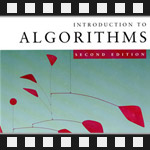This is the tenth post in an article series about MIT's lecture course "Introduction to Algorithms." In this post I will review lecture fifteen, which introduces the concept of Dynamic Programming and applies it to the Longest Common Subsequence problem.

Dynamic programming is a design technique similar to divide and conquer. Divide-and-conquer algorithms partition the problem into independent subproblems, solve the subproblems recursively, and then combine their solutions to solve the original problem. Dynamic programming is applicable when the subproblems are not independent, that is, when subproblems share subsubproblems. A dynamic-programming algorithm solves every subsubproblem just once and then saves its answer in a table, thereby avoiding the work of recomputing the answer every time the subsubproblem is encountered.

Dynamic programming was systematized by Richard E. Bellman. He began the systematic study of dynamic programming in 1955. The word "programming," both here and in linear programming, refers to the use of a tabular solution method and not to writing computer code.

Dynamic programming is typically applied to optimization problems. In such problems there can be many possible solutions. Each solution has a value, and we want to find a solution with the optimal (minimum or maximum) value. We call such a solution an optimal solution, as opposed to the optimal solution, since there may be several solutions that achieve the optimal value.

Dynamic programming can be effectively applied to solve the longest common subsequence (LCS) problem. The problem is stated as following: given two sequences (or strings) x and y find a maximum-length common subsequence (substring) of x and y.

For example, given two sequences x = "ABCBDAB" and y = "BDCABA", the LCS(x, y) = { "BCBA", "BDAB", "BCAB" }. As you can see there are several optimal solutions.

Lecture fifteen introduces dynamic programming via this longest common subsequence problem. It first gives a brute-force, exponential time algorithm for solving it. The idea of algorithm is to check every subequence of x[1..m] (m is the length of sequence x) to see if it is also a subsequence of y[1..n] (n is the length of sequence y). Checking takes O(n) time, and there are 2m subsequences of x. The running time thus is exponential O(n·2m). It is no good for large sequences and the lecture continues with a simplification - let's look at the length of a longest-common subseq and then extend this algorithm to find the LCS itself. The simplified algorithm is recursive in nature and computes the same subproblems. At this moment two dynamic programming hallmarks are stated:

• 1. Optimal substructure: an optimal solution to a problem contains optimal solutions to subproblems.
• 2. Overlapping subproblems: a recursive solution contains a "small" number of distinct subproblems repeated many times.

As the subproblems are overlapping, the lecture introduces concept of memoization algorithm (note that it's not memorization). A better known word for memoization is caching. The subproblems are cached (memoized) so that they are not recomputed over and over again.

The lecture ends with constructing a dynamic programming table for LCS problem and explains how to find a LCS from this table.

You're welcome to watch lecture fifteen:

Topics covered in lecture fifteen:

• [00:20] Dynamic programming.
• [01:47] Longest common subsequence (LCS) problem.
• [03:55] Example of LCS on sequences "ABCBDAB" and "BDCABA".
• [06:55] Brute force algorithm for LCS.
• [07:50] Analysis of brute force algorithm.
• [11:40] Simplification of LCS problem.
• [16:20] Theorem about LCS length.
• [18:25] Proof of the theorem.
• [30:40] Dynamic programming hallmark #1: Optimal substructure.
• [32:25] Example of hallmark #1 on LCS.
• [34:15] Recursive algorithm for longest common subseq.
• [36:40] Worst case analysis of the algorithm.
• [38:10] Recursion tree of algorithm.
• [42:40] Dynamic programming hallmark #2: Overlapping subproblems.
• [44:40] Example of hallmark #2 on LCS.
• [45:50] Memoization algorithm for LCS.
• [48:45] Time and space analysis of memoized algorithm.
• [54:30] Dynamic programming algorithm for LCS.
• [01:01:15] Analysis of dynamic programming algorithm.

Lecture fifteen notes:

This course is taught from the CLRS book, also called "Introduction to Algorithms". Chapter 15 is called "Dynamic Programming" and covers the topics in this lecture. It also explains the assembly-line scheduling problem, matrix-chain multiplication problem, elements of dynamic programming and optimal binary search trees.

The next post will be about graphs, greedy algorithms and minimum spanning trees.